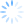Up to this point we’ve focused primarily about how to use Visual Basic in Microsoft Excel to programmatically access the functionality of our ZIP code and location analysis tool, CDXZipStream.  (Please refer to our previous posts Calling CDXZipStream with Visual Basic – Part I and Part II.)  Now it’s time to do the same for Microsoft Access.  Side note:  The form of Visual Basic when implemented in Microsoft Office products is referred to as Visual Basic for Applications, or VBA.  We’ll use these terms here interchangeably.

The approach to accessing CDXZipStream functions is very similar for Access and Excel, although there are obviously significant differences in how the input and output data are handled since the data resides in database tables versus worksheets.  So let’s start out with the same simple example that we used previously for the VBA code for Excel, where we request city information for a given ZIP code.  The CDXZipStream formula is:

= CDXZipCode ("07869", "City")

In a Visual Basic module we use the CreateObject statement to connect to CDXZipStream:

Then simply ask for the data:

To loop through records in a table and return city information for a list of ZIP codes, here’s how it would look:

Sub GetCityData()

Dim db As Database

Dim rs As Recordset

Set db = CurrentDb

Set rs = db.OpenRecordset("Select * from MyTable")

Do Until rs.EOF

rs.Edit

rs.Update

rs.MoveNext

Loop

End Sub

The code assumes that the Access database contains a table called MyTable that includes at least two text fields, ZIPCode and City.

Let’s also look at a more complex example where both the input and output data are in the form of arrays, as when optimizing stops in a driving route.  Let’s say we have a table that contains up to five input addresses per record, with each record representing a driving route.  We want to determine the order of the addresses that result in the quickest driving time, and output the new order of addresses to the same table.  The code below loops through each record, to optimize all driving routes in the table:

Sub GetOptimizedRoute()

Dim db As Database

Dim rs As Recordset

Dim InputArray As Variant

Dim OutputArray As Variant

Set db = CurrentDb

Set rs = db.OpenRecordset("Select * from MyTable")

Do Until rs.EOF = True

'Count the number of addresses in the input array

InputArray_cnt = 0

For I = 1 To 5

If IsNull(rs.Fields("Address" & I & "")) = False Then InputArray_cnt = InputArray_cnt + 1

Next I

'Optimization requires at least 4 addresss

If InputArray_cnt < 4 Then GoTo Skip_record

'Dimension the input array according to previous count

ReDim InputArray(InputArray_cnt - 1)

'Populate the input array, while skipping fields with no data

X = 0

For I = 1 To 5

If IsNull(rs.Fields("Address" & I & "")) = False Then

InputArray(X) = rs.Fields("Address" & I & "")

X = X + 1

End If

Next I

' 0 is the parameter that specifies the quickest route

' 8 is the parameter that specifies the output as an array of addresses in optimized order

' Put the output array results in the output address fields

If IsArray(OutputArray) = True Then

For I = 1 To InputArray_cnt

rs.Edit

rs.Fields("Address" & I & "_opt") = OutputArray(I - 1, 0)

rs.Update

Next I

'If a non-array result is returned due to error, send the result to the first address field

Else:

rs.Edit

rs.Update

End If

Skip_record:

rs.MoveNext

Loop

End Sub

MyTable here contains input fields Address1 through Address5, and output fields Address1_opt through Address5_opt; the latter addresses are listed in their optimized order.  Not all the input address fields need to populated, and for real world examples it would be easy to add fields as necessary to accommodate many more address stops on a route.  However, a minimum of four addresses must be input for optimization to proceed.

Note: You can copy and paste the code here to test it in an Access VBA module, although it may require some modification to fit your particular needs.  Don't forget to include the Option Compare Database statement at the top of each module!